## Saturday, August 23, 2008

### Untangling with Continued Fractions: Part 2

Recall that I defined a rational tangle to be what you get by starting with a pair of untangled strings whose ends are at infinity and then sliding the strings around as much as you like keeping the ends at infinity. If you don't like the infinity bit, just think of strings inside a sphere with the ends constrained to lie on (but free to slide around) the surface of the sphere. We also insist that the ends end up at the four diagonal directions.

Two rational tangles are considered to be isotopic if you can slide the strings about to turn one into the other, this time keeping the ends of the strings fixed. (Such a motion is called an isotopy.)

As I've previously mentioned, you only need twists, antitwists and rotations to untangle a rational tangle. Here are the twist operations again: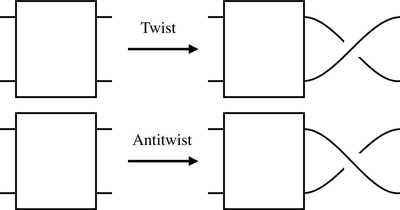I'm using a box to represent some unknown tangle. The antitwist really is an inverse for the twist because one followed by the other gives you what you started with once you pull the strings straight:We can also draw a rotation like this: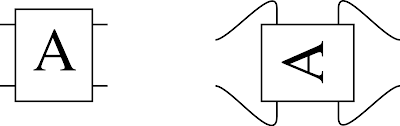Let's call the twist T (so the antitwist is T-1) and the rotation S and write products of operations from right to left in the usual way. As both S and T are invertible, they generate a group. It's pretty clear that S4=1, where 1 is the identity operation. So we don't need a clockwise rotation, we can just use S3.

But here's a surprising fact: S2=1. If we perform a 180 degree rotation on a rational tangle the result might look different at first, but it is isotopic to what we started with. I'll leave you to convince yourself of this, but you can prove it by induction on the number of twists or antitwists you have in your diagram. Try it for simple rational tangles first, maybe real ones made with real string.

Here's an even more surprising fact, (TS)3=1. I can demonstrate this pictorially. Start with he result of applying T: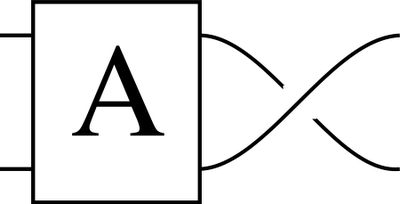The result of ST:The result of TST: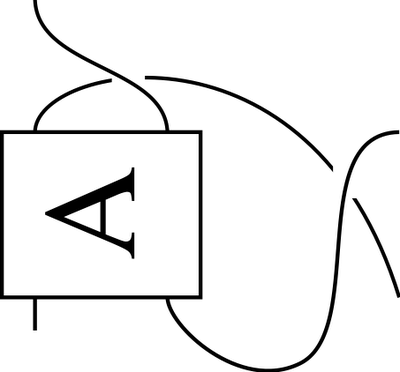The result of STST: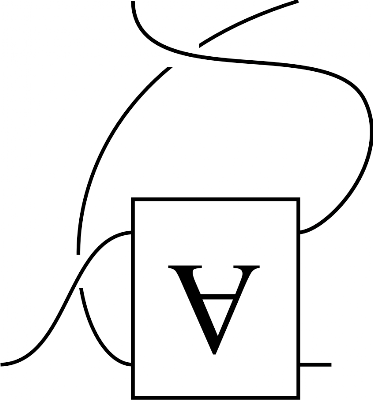After TSTST:But by sliding one string behind the tangle, allowed in an isotopy, we find that that is isotopic to this:It's not hard to see that another S brings us back to where we started. (To do that we need to rotate the block labelled A, but that is allowed in an isotopy as we're not moving the ends of the strings.) This means we can write an antitwist in terms of twists and rotations. But it's convenient to keep using antitwists.

So we have S2=1 and (ST)3=1. It can also be proved that any other relationships between S and T can be derived from these using the operations in a group. But this is a well studied group. We can approach it like this. Define two functions s and t by

s(x) = -1/x

t(x) = x+1.

It's easy to show that s2=identity=(st)3. In fact, we have an isomorphism between the group of operations we can perform on rational tangles and the functions we can build with s and t. It goes further.

Take the rational numbers and throw in ∞ to give us the extended rationals. Let s and t act in the usual way on most of the rationals but throw in the extra rules s(∞)=0, s(0)=∞ and t(∞)=∞ to handle infinity. We have defined an action of s and t on the extended rationals. We can exactly parallel this with the rational tangles. Call this tangle 0:We can reach every rational tangle by applying the operations S and T to 0. But if we replace S with s and T with t then the sequence of s's and t's will give us an extended rational number that labels the tangle. Because S and T obey the same equations as s and t, isotopic knots will end up getting the same extended rational. So we can use extended rationals to label the rational tangles. Here are some examples:

Firstly just an antiwist, T-1. That corresponds to t-1(0)=-1.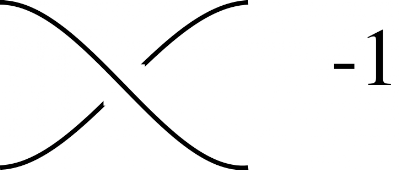Two antitwists gives -2: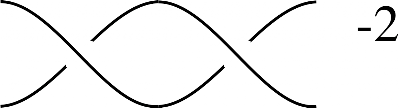Composing a 90 degree rotation gives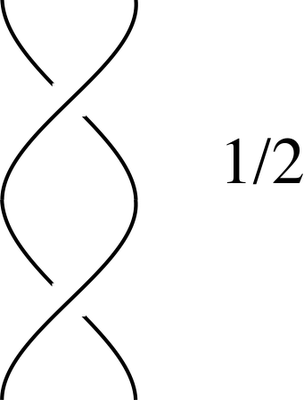And applying another antitwist subtracts one again giving:The name rational tangle is justified.

So, given any any rational tangle we simply need to find the corresponding extended rational and then figure out how to write it as a composition of s's and t's applied to 0. This is more or less the usual technique for finding the continued fraction representation of a rational. And once we know the sequence, we can then apply it in reverse to untangle the tangle.

But there's one catch, how can we go from the monadic representation of a tangle that I described previously to the corresponding extended rational? We'll start on that problem in my next post, but amazingly the vector space monad can be used to do almost all of the work. It's surprising: it's only a short bit of code that works out the untangling operations, but the explication is getting rather long now...

An amazing application of this work is in the study of the untangling of DNA. DNA is a double helix and the two parts of the helix must be separated in order to transcribe it. As you might imagine, the DNA can become incredibly tangled by this process. To deal with this, the topoisomerases snip, manipulate and repair the DNA so as to eliminate these tangles. It was realised that it was possible to work out exactly what operations the topoisomerases were performing by analysing the before and after DNA strings in terms of rational tangles.

The perosn who first figured out this relationship between tangles and rational numbers was the ubiquitous JH Conway.

### References

For the proofs missing above, these references should fill in the details:
Rational Tangles, Kauffman and Goldman
Knots and Physics, Kauffman
And if I've lost you above, the following is a nice easy introduction:
Conway's Rational Tangles, Davis
Modeling protein-DNA complexes with tangles

One last thing. I've noticed some, but not all, papers define the twist oppositely from me.Anonymous said...

The plan to figure out how to get the extended rational by applying s and t to 0 seems to imply that the stabilizer of 0 is trivial. This is not the case, STS also stabilizes 0. There must be something you're still hiding from us. Maybe the whole stabilizer is the group generated by STS, so the plan gives a way to go to a boring tangle with extended rational 0 which is easy to untangle.
Anyway, fascinating stuff, I'm curious how the story continues.

Sunday, 24 August, 2008sigfpe said...

I'm not sure what I've misled you to believe. I'm not claiming a 1-1 correspondence between the elements of our group and the rational tangles so it's fine for STS0=0 (and sts0=0).

Sunday, 24 August, 2008Anonymous said...

It's the paragraph
So, given any [only now that I'm citing it I see that you have that little word twice] rational tangle we simply need to find the corresponding extended rational and then figure out how to write it as a composition of s's and t's applied to 0. [...] And once we know the sequence, we can then apply it in reverse to untangle the tangle.

This could only work completely if there were a 1-1 correspondence. Maybe the trick is that it works good enough, i.e. gives an easily untangled tangle.

Sunday, 24 August, 2008sigfpe said...

Call the group of twists and rotations G. Call the group generated by s and t, g. There is an isomorphism, call it p:G->g. Call the set of rational tangles (up to isotopy) K. Call the extended rationals k. There is a 1-1 correspondence between these sets; call it q:K->k.

Not only is p a homomorphism between groups but the action of the groups on their respective sets is compatible with p and q.

So if x is in G then not only is p(xy)=p(x)p(y) etc. we also have that for any rational tangle t in K, q(xt)=p(x)q(t).

There is also a map G->K taking x to x0. Every rational tangle is of the form x0 for some x in G so this map is surjective. But it's not an isomorphism. Maybe when I said "Once we know the sequence..." I gave the impression that it was a unique sequence, but I was just using 'the' to refer to the composition in the previous sentence. The extended rational however is unique, there is precisely one for each rational tangle even though there are multiple ways to build this rational using s and t from 0.

I'm probably completely missing what you're saying, but the above should disambiguate a lot.

I do claim that the sequence of s and t's should take you all the way back to precisely 0.

Sunday, 24 August, 2008Anonymous said...

I was still expecting G and K to be essentially the same (as moves on rubik's cube and positions on it), and was doubting that q was 1-1.
Now I realize that in your first comment, when you wrote STS0=0, you meant the 0 in K, not k (where it acts on via p). (Of course it doesn't matter much when q is 1-1.)

My intuition doesn't work here, so I guess I should follow the references and take a look at the exact definitions.

Sunday, 24 August, 2008geophf said...

*sigh* I've been repressing my fascination with knot theory since I was introduced to the concept way back in college. But your articles have forced me now to purchase Formal Knot Theory, by Kauffman. The thought of the applications of this theory are bewitching! But that's just drop of the ocean of pleasure to be had at cocktail parties:

Jrandom: "So, what do you do?"
Me: "Oh, I'm a Knot theorist by profession. How about you?"
Jrandom: ... response silenced by sounds of choking on the salmon mousse canapé.

You also mentioned a few posts ago that you have a LaTeX to Blog/HTML system you built. Would you be willing to share the source code? Or, to make a literate Haskell source entry of it on your blog?

Sunday, 24 August, 2008Anonymous said...

Finally, everything makes sense!
And I didn't have to read more than what you wrote.

The definition of 0 (in K) was so unsurprising, and my misguided intuition told me that directions weren't important, that when thinking about it and when eventually doing real physical experiments, I was starting with S0 instead of 0 without noticing.

Now I can see STS0=0, and with a bit more effort TST0=0.
Using correct definitions really helps...
Sorry for the confusion.

I'm still wondering whether the cyclic group generated by STS is the whole stabilizer of 0.

Monday, 25 August, 2008Anonymous said...

How the fraction will happen if we are using 3 strings?

Wednesday, 27 August, 2008